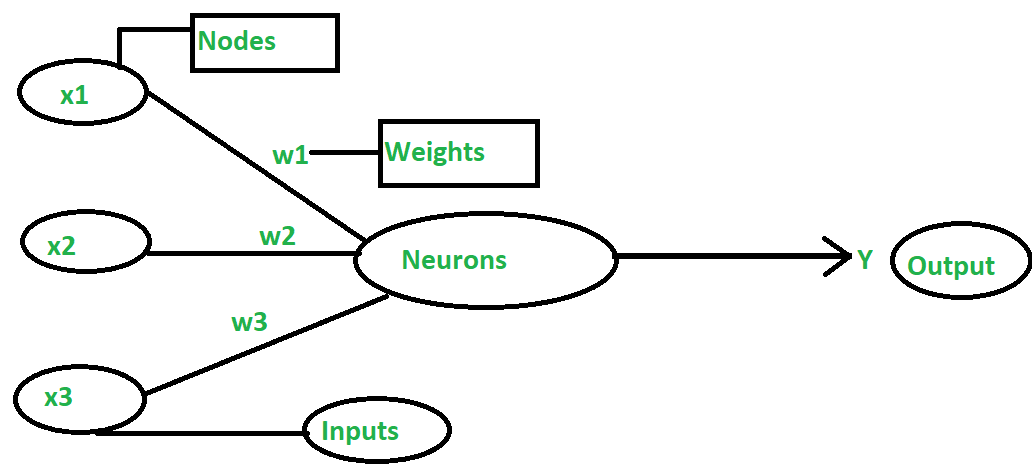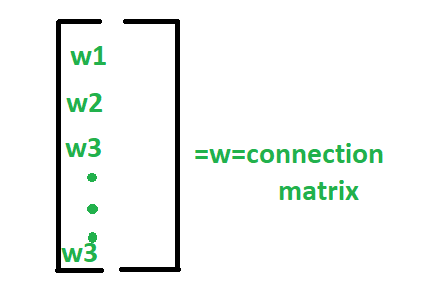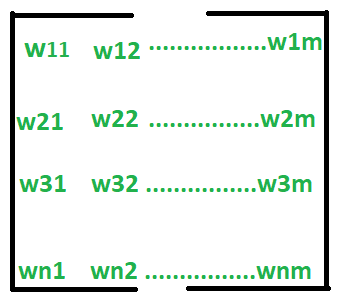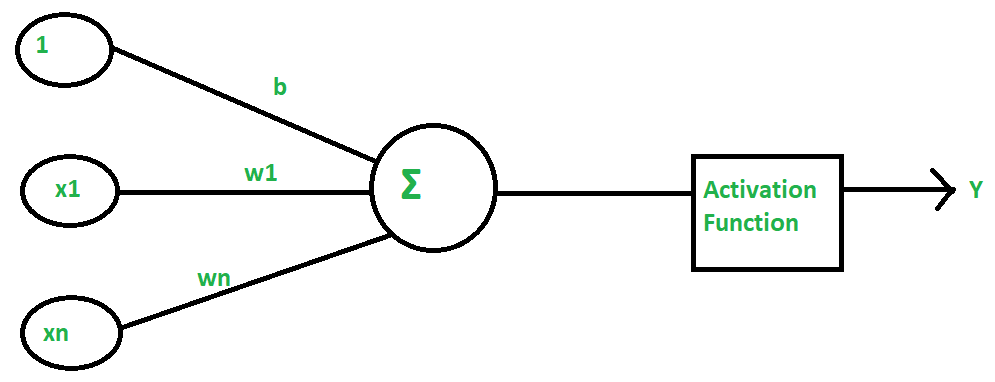# Artificial Neural Network Terminologies

• Last Updated : 05 Apr, 2022

The ANN(Artificial Neural Network) is based on BNN(Biological Neural Network) as its primary goal is to fully imitate the Human Brain and its functions. Similar to the brain having neurons interlinked to each other, the ANN also has neurons that are linked to each other in various layers of the networks which are known as nodes.The ANN learns through various learning algorithms that are described as supervised or unsupervised learning.

• In supervised learning algorithms, the target values are labeled. Its goal is to try to reduce the error between the desired output (target) and the actual output for optimization. Here, a supervisor is present.
• In unsupervised learning algorithms, the target values are not labeled and the network learns by itself by identifying the patterns through repeated trials and experiments.

### ANN Terminology:

• Weights: each neuron is linked to the other neurons through connection links that carry weight. The weight has information and data about the input signal. The output depends solely on the weights and input signal. The weights can be presented in a matrix form that is known as the Connection matrix.•  if there are “n” nodes with each node having “m” weights, then it is represented as:• Bias:  Bias is a constant that is added to the product of inputs and weights to calculate the product. It is used to shift the result to the positive or negative side. The net input weight is increased by a positive bias while The net input weight is decreased by a negative bias.Here,{1,x1…xn} are the inputs, and the output (Y) neurons will be computed by the function g(x) which sums up all the input and adds bias to it.

```g(x)=∑xi+b where i=0 to n
= x1+........+xn+b```

and the role of the activation is to provide the output depending on the results of the summation function:

```Y=1 if g(x)>=0
Y=0 else```
• Threshold: A threshold value is a constant value that is compared to the net input to get the output. The activation function is defined based on the threshold value to calculate the output.
```For Example:
Y=1 if net input>=threshold
Y=0 else```
• Learning Rate: The learning rate is denoted α. It ranges from 0 to 1. It is used for balancing weights during the learning of ANN.
• Target value: Target values are Correct values of the output variable and are also known as just targets.
• Error: It is the inaccuracy of predicted output values compared to Target Values.

Supervised Learning Algorithms:

• Delta Learning: It was introduced by Bernard Widrow and Marcian Hoff and is also known as Least Mean Square Method. It reduces the error over the entire learning and training process. In order to minimize error, it follows the gradient descent method in which the Activation Function continues forever.
• Outstar Learning: It was first proposed by Grossberg in 1976, where we use the concept that a Neural Network is arranged in layers, and weights connected through a particular node should be equal to the desired output resulting in neurons that are connected with those weights.

Unsupervised Learning Algorithms:

• Hebbian Learning: It was proposed by Hebb in 1949 to improve the weights of nodes in a network. The change in weight is based on input, output, and learning rate. the transpose of the output is needed for weight adjustment.
• Competitive Learning: It is a winner takes all strategy. Here, when an input pattern is sent to the network, all the neurons in the layer compete with each other to represent the input pattern, the winner gets the output as 1 and all the others 0, and only the winning neurons have weight adjustments.
My Personal Notes arrow_drop_up